THE  g/  FACTOR

CHAPTER 60     (Nov 2010)INDEX TO OTHER PAGES

1. In a continuation from page 58 (factors of gravity) there is more to consider, By illustration figure 60-1, if we were on a space ship traveling in a straight line between the earth and the moon, and we reckoned our inertial value of the A/factor by our earthly standards, with the L/factor at 25,000 mph, will that be the sum of the inertia?

2. Or, are we perhaps forgetting that we have an additional L/factor driving us at the velocity of 15 Km/sec, at a right angle?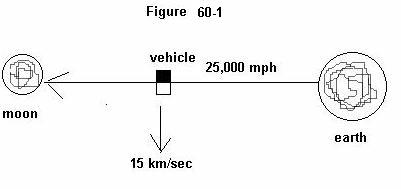1. No doubt all things are relative, but we ought to have a closer look at ourselves upon mother earth by an illustration figure 60-2. We found it factual that at sea level we are drawn to the earth by a gravitational pull of 32.174 (in lb).

2. And we found it factual by our laws of motion into the centrifugal, that this factor of gravitational pull - by the velocity in which it is driven linearly, effects a resistance that by measure in force is squared. Like when a vehicle plows into a brick wall the force by velocity becomes four-fold.

3. The same thing occurs when we take a turn upon the road, and again when we place ourselves in a uniform circular motion, such as we are doing when located anywhere upon the earth excepting the very tips of the earth's axis, where we may be free of that centrifugal impact.

4. And for the example by figure 60-2, taking a mass of 500 lb., which when located at point D, upon the axis of the earth on a scale will read 500 lb.

5. Then by placing it at point E, the earth's equator, it continues to read 500 lb., yet now we are 4000 miles removed from that axis traveling at near 1000 mph in a uniform circular movement, which as such effects a push into the centrifugal, meaning away from the surface of the earth.

6. And yet at either point E, or D, we did not move, nor did we notice any such push, nor did we read its effect upon the scale.

The question then becomes how is that so?

1. For by law there is an inconsistency in the measure of the force of gravity at point E which must come to 552.2 lb. If not, we might as well scrap our laws of motion, and/or that factor of gravity which we found by acceleration.

2. Are we then saying that the force that holds us to the earth has no effect upon any centrifugal implications?  But that can-not be true - can it?

3. For we know for a fact that at high speed upon a road, and attempting to take a turn - our movement tends to keep us into our straight trajectory. And we know that by the centrifugal impact the earth bulges somewhat at its equator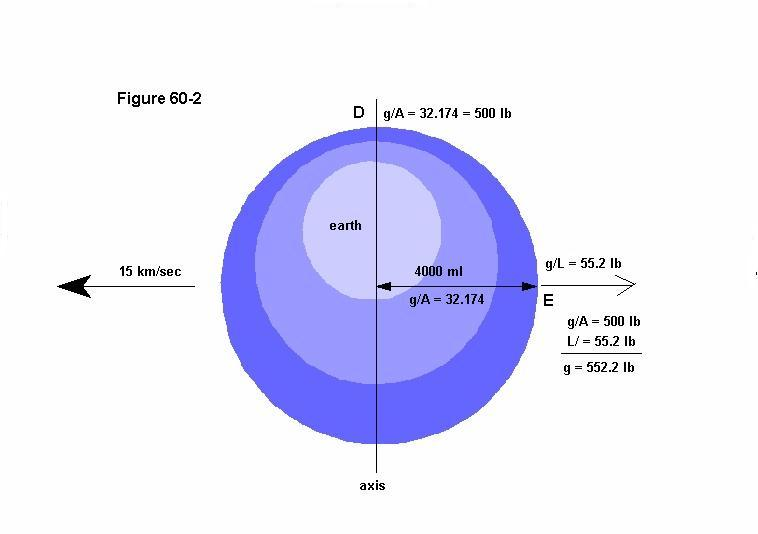Click on picture for larger view.

1. So why then at the speed of 1000 mph, (as fast as a jet airplane) do we keep our feet upon the ground rather than being driven in a straight trajectory away from the surface of the earth?

2. It has to be true and correct that our force of gravity places a due hold upon us in that measure of 55.2 lb., just as it does upon any space shuttle or those many satellites we have circling our earth.

3. Mother earth then for its inborn gravitational pull will always show us that same measure of itself affixed to 32.174, with these exceptions where at its equator, it is somewhat bulged - due to that high centrifugal impact upon it creating a bulge showing us a variation of its inborn force of one half of a pound.

4. And of course that also show how that amount of pull is in part relevant to distance from the center.

5. To then double check our reasoning, it is surely correct that upon the scale the centrifugal measure of 52.2 lb, for a mass of 500 is not read, yet it did read that minor one half of a pound.

6. Thus we must discover how and why this is so, - since - as sure as we are of the first, we are equally certain of this that a centrifugal impact effects a gravitational draw upon us.  And so what might that be?

7. Shall we place it in the nomenclature of the earth, that molten mass which effects a strong magnetic force, that by the inertia of anything located at its surface affixes a set measure of gravitational draw, which can-not be changed, lest its internal nomenclature changes, after which it draws on all mass that is effected by the linear?  For here we have come to an interested aspect to perhaps solve our problem.

8. I now could simply state the how so, and the why, but you also must be able to reason it out in yourselves, wherefore I am effecting learning along with revelation.

9. And in order to keep you with me in this reasoning, we must beforehand refer to several other illustrations, beginning with figure 60-3 to make sure that we understand the mechanics of how the inertia of all matter works, and how earth's magnetic entity picks up on it to hold it in its embrace.

10. The cause for the half pound difference from pole to equator is not by virtue of the centrifugal.  Since that reading of it has no bearing at all on the axis of the earth, but rather to the magnetic force of the earth inborn within it that acts by its volume.  And since that volume, or distance is a bit more at the equator we come with a half pound difference between it and the polar regions.

11. The atoms then of all substance being effectively miniature gyro's, is what we came to call the A/factor, the angular movement, that as such being a movement that comes back to the same point, and affixes itself to resist any change in movement as well as displacement.  But when it is forced into a linear displacement, it resisting the change, begins to precess.

12. By the illustration at C, the torque is the the L/factor, promoting that precession A to B, which then becomes susceptible the the magnetic embrace of the earth, in the embracement drawing the very atom to itself.click on picture for full view

1.  Wherefore it is important to remember that a gravitational movement does not proceed unless there is a torque upon it, (also said; unless there is movement into the linear)  after which it essentially screws itself down by virtue of the magnetic lines of movement.

2. And to go one step further in what manner the 3M (magnetic motion) actually draws upon all substance. It is not like grabbing a hold of the bottom and pulling, but for its full embracement it is more like going around it - and push/pulling on it.

3. This may be illustrated by figure 60-4, where the 3M coming out of the earth's surface passes over the mass, not only from one direction in the loop but from both directions.

4. This may seem new to us in that when we look at the illustrations of the magnetic force coming from the earth, the movement in direction is going in at the south, and coming out at the north.

5. This however in my view is the "overall" direction of it, while at fundamental levels it is in both directions at either or both poles, as it must be for its fundamental hold.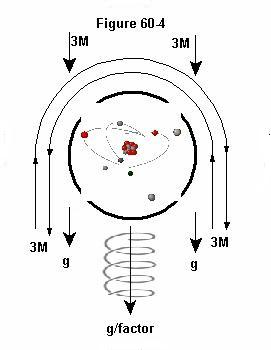1. It is for that reason that in figure 60-3, I drew a lead/lag scenario by which anything into an angular precession also moves into the linear, the screw of a propeller giving a likeness thereto. And for more confirmation look at the picture of the sun here how that flare of the sun displays a loop.

2. That sun-flare then in its loop is in an overall scheme of things, but the same is correct at fundamental levels. The lines of that sun-flare are in effect the magnetic lines of movement, embracing the fiery substance holding it by what we call gravitational, but those circular loops are clearly magnetic lines.

3. Now we are to come back to illustration figure 60-2 again, and our 500 lb mass which at the equator refused to show itself for the full force of gravity upon it.

4. We might therefore say that the first draw of gravity, the 500lb., by its 32.174 measure - by and of the "angular," the A/factor, while the second, the 55.2 lb., was added by the "linear," the L/factor.

5. I wrote on this many years ago in page 28 of this website, but not as detailed in a fashion of learning as we are doing now. We are however by the foregoing not home yet, claiming that the inborn gravitational pull is only upon the A/factor.

6. It seems to be logical, for while the earth moves swiftly in space and displays a g/force of a fixed measure, the very 24 hour rotation of the earth does not seem to add anything to it, obvious from the fact that the 500 lb., mass remained at 500 lb., at both points D, and E, and we had to get our calculator out in order to discover what the additional g/force might be at any place other than the tip of the axis.

7. But did I not here previously mention something important, to wit, how for a gravitational pull to set in, there had to be a torque upon that angular, a torque which usually comes about by a linear force unto it?

8. How then might it be only affixed upon the angular factor? And what are we to conclude for that 15 km/sec orbital movement which the whole earth makes? Movement after all is movement in any fashion.

9. And yes we should conclude that our 15 km/sec orbital motion is effectively an L/factor upon all the atoms of any earthly mass?

10. But why then is the same not compounded with the L/factor of our 24 hour rotation? The inertia of the mass of our earth seems to be well fixed in its orbital movement for a force to promote fundamental precession, and the power of its magnetic fabric thereupon.

11. Then there is this to further confuse us where many years ago, in page 28, I wrote; quote:  "The statistics here are;  g force, or centripetal force is 235.51-lb., gravitational weight is 163.3- lb., spring weight is 163.32-lb., centrifugal inertia is 72.19-lb.

12. The reason that now his gravitational and spring weight are the same, is because we set our standard of weight free of centrifugal inertia, otherwise weight would vary from equator to pole.  And it is verified since the additional 1521-ft/sec in his velocity took away only 1.68-lb. of his spring weight, yet it took 2.25-lb. of his gravitational weight.

13. If then we traveled some 339 mi/hr more than we are, or the earth made one revolution in a little more than 18 hours our gravitational and spring weight would be equal since:

14. 165 x 2019-ft/sec2 : 20929920-r = 32.14 : 32.14 =1.   For in that case our velocity in force comes equal to the force of the gravitational acceleration.  (unquote)

RETURNING

1. Now so many years later I came to wonder how I got to this. But it is simply so that at a greater rotational velocity our weight upon the scale comes equal to the affixed rate of 32.174. But that, as I surmise, is only at any one latitude upon the earth, the scale to vary everywhere else.

2. Or again where I wrote:  "This shows that there is no radius, nor speed of any mass in or out of orbit to enter into a calculation of weight as a measure of gravitational force.  And also to reveal that all linear inertia is incidental, a separate cause, a force into the centrifugal, not of gravity.

3. But that the same is counteracted by the gravitational force in a measure ounce for ounce (one on one) to the limit of g - where the first angular inertia is overcome (exceeded into) the linear inertia.

4. Consequently, if inertia were a single component, our weight upon the earth would vary from equator to pole, and we would not be able to fly an SR-71, nor venture out in to space short of being crushed by our own weight.

5. And angular inertia, as no more and no less than the angular momentum of each single atom and the quantity of them in any single mass (upon which gravitational force bears down, or attaches itself), is as such distinct from the linear inertia by velocity. Unquote.

6. Thus I promoted a distinction in how gravitational acts upon the one and the other, the linear verses the angular.

7. Then by illustration figure 60-5, we will first assume our earth at a stand still, and to place a force upon it at point H, to drive it away in a straight on trajectory towards M to the tune of 15 km/sec.  Then we go back to basics to behold how that mass of atoms of the earth reacts to this acceleration.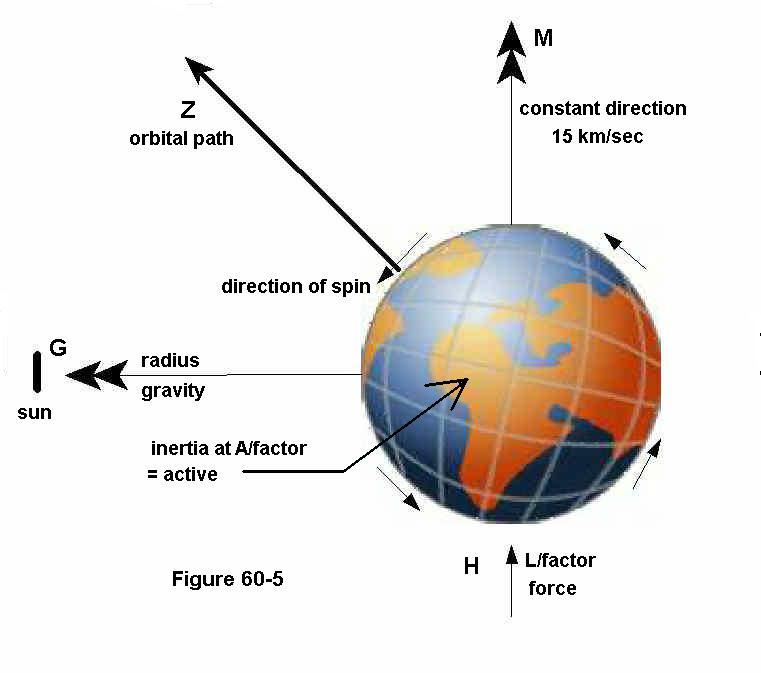click on picture for full view

1. All that angular movement of the fundamental parts of any mass have this tendency, to resist being moved, and once in motion to resist being stopped again, as well to resist any change in direction.

2. Thus with the earth at an idle the angular momentum of its mass may be likened to what is presented in figure 60-6 at Q.  The A/factor is active, but the L/factor is at zero, while the atomic gyro's are at what we may call in an upright state of balance.

3. Then as we began to move the earth to accelerate it, - the L/factor stepped in, placing a torque upon that angular motion, by illustration location R, pushing the top of the gyro over causing it to precess.

4. Then as in figure 60-5 we reached our maximum velocity of 15 km/sec, the force at figure 60-5 -H, is abandoned, while the earth continues to move at its now constant velocity in a straight trajectory.

5. At this point there is not as yet an vector, nor therefore an orbit.   And with the force at 60-5-H, gone, the L/factor at R, in figure 60-6 has likewise left off, and comes back as were it at Q. The precession seen at R  (for the spinning top) will however remain, for once something set in motion, or into a direction of motion it will continue so, unless acted upon otherwise.

6. This then is the elementary view of it, a bit simpler to understand than the in-depth scenario, at which we shall come back another time. This however is correct that at this point there is no duress, not any torque upon it, the T/factor left off as soon as the L/factor left off.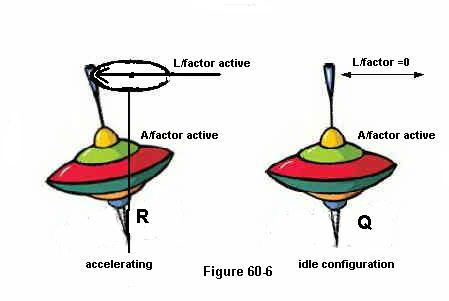1. Thus as we visualize that earth now without any power acting on it happily moving at its said velocity, the only real action occurring within at fundamental levels is the A/factor, the atomic angular movement that can never be removed.

2. Yet it does have a degree of overall precession upon its general movement. This last part though it is not an inclination towards gravity, it is nonetheless a factor upon which the 3M, the magnetic is susceptible.

3. And to rehash this, at Q in figure 60-6 with the atoms at near upright balance there is no application for the magnetic entity to lay any real hold upon it, although susceptible, while at R, - it - not as yet taking a hold for a movement into the gravitational, found by the format by which it might eventually draw it into the gravitational.

4. "Once in motion stays in motion," and; "Once in direction, stays in direction," are thus very correct. The earth at this point has no inclination to slow down, for even as much as it resisted the acceleration, it equally resists any deceleration.

5. And while thus far there was not any magnetic force to be found, the earth continued on its straight trajectory oblivious to anything, nor therefore any gravity. It is no more than a mass moving in space.

6. For yes I am doing this in a step by step procedure to not only reveal physics, but to teach the same as well, for it is written: "God of the sciences and of learning."

7.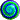Then let us create that circular movement which when twisted by 180 degrees over itself becomes what we call - "magnetic."  Not that we are so competent, but the Almighty Lord did so in His in-measurable wisdom.

8. But to secure a magnetic entity one needs a body to implement it upon, which as such then becomes a vector.  And for our first consideration, let it be the sun, at point G, in figure 60-5 so that we might make some use of that mass in space, to make it into an earth, and not forever go to the far reaches of the universe. To in other words create a home for ourselves, keeping it in place.

9. The 3M of that sun then looks at our A/factor and observes a precession of it with no torque. And for its particular nature of movement being susceptible to that precession it embraces it.

10. If then we think, Okay it has a good hold of us, we are in error.  Up to this instant, having the earth in its embracement - there is not sufficient power to divert it from its direction of movement at the velocity of 15 km/sec, at which it travels.

11. The A/factor at its balanced state with no torque is sufficient inertia to keep it going and to resist any change in velocity, but equally as much to divert it from its direction, wherefore unless something occurs by which the sun in its 3M may increase its power upon it, the earth will not find a home.

12. That occurrence then is simply a factor of movement, as all things come about by movement.

13. Visualize this: Here is the earth happily moving, and just now we latched onto it with a rope called 3M. The instant then when we do so - that earth being in motion, our rope may be stretched, or snap loose altogether slipping through the mass of the earth, because the A/factor is without torque, and a mere balanced state is no way to implement a good knot upon it.

14. Then we come to figure 60-7, to see what occurs at those fundamental levels. At K, the A/factor is presented for a constant balanced ideal having no torque upon it. And as such that format here drawn by an oval is merely to depict a coordinate, not the likes of a wave, but assuredly a pattern by which movement follows.

15. Then as the earth attempts to get away from our 3M rope, that rope - as we might say - tightens around that precession in the pattern thereof, implementing a torque upon it, a torque in strength equal to that of the earth in its velocity relative to its vector in radius, as presented by figure 60-7 -S.

16. These are fancy words, simply to say that - the greater the distance is from the vector so much the smaller the deviation needs to be for the earth to come out of its linear trajectory.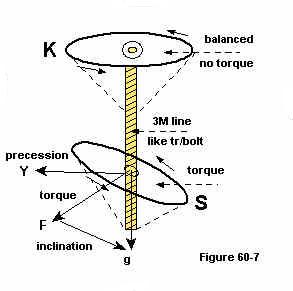1. If the radius was 46 million miles instead of the 93 million, the torque implemented would be twice as great in order to maintain it in a uniform circular movement.

2. In the final outcome thus - it is the quantity of the inertia relative to speed and radius. Or in more fundamental terms, the degree of torque upon the A/factor relative to the L/factor in the mass volume thereof.

CREATING 3M

1. Now that we have our earth bound to the sun at a yearly clip around it, the next essential factor is to create a magnetic entity upon the earth so that it may take on the shape of a sphere.  For in placing a magnetic entity on it, it likewise, like the sun at first, - is susceptible to the very same angular precessional movement, and as such holds it in place.

2. But here, there is a distinct difference in that the former magnetic vector was 93 million miles away with only its tentacles holding us, that now it is seated right upon that very mass itself.

3. It then centers itself upon that mass by virtue of its own design, for while being movement - it is after all a coordinate that embraces all of the inertial mass, nor can it therefore be more to the one side and less to the other, lest it be off balance in itself.

4. Remembering how that coordinate is in all respects a figure of eight, and the movement of it as it passes through the earth is by a figure of eight. (Magnets also when broken in half reseat themselves on their new centers, and likewise with unstable atoms.)

5. Now then being seated upon all that angular inertia, it embraces it in what is essentially the balanced configuration of that angular inertia, like unto K, in figure 60-7.   Then we are to pay good attention to what comes next.

6. That well seated magnetic entity does not need a torque in order to hold onto all of that volume of the earth-mass, the precessional inclination of the A/factor is sufficient,

7. since the mass as such is not attempting to depart from its center, or vector if you will. The whole earth simply becomes like a single magnet, with a factor of magnetic pull that at sea level is in the amount of 32.174 (in lb)

8. Are you still with me so far, or am I going too fast?  For we were assuming the earth as a none rotating body, nor am I in error about stating the 32.174 being a factor of "magnetic" pull.

9. Note therefore how I changed the term "gravity" into "magnetic", and I did so to make us think, and to make us correlate one factor with another, since gravity is a lady with magnetic her man.

10. And not man was made from the woman but the woman from the man, so gravity comes forth from and by magnetic in conjunction with the inertia of the mass within its embrace.

11. Accordingly there is now a force of gravity upon the earth that can be read upon any scale, while the earth has not as yet a period of rotation.

12. If now one will question me; how if the earth has its own draw, how are we held to it that are not part of the earth, but simply walking upon it?  The answer here is, that we in fact are a part of that very earth. In that respect the whole earth with everything that is upon it, is as were it a single entity vectored to the center of eight.

13.Now then we are going to give the earth a period of rotation.  But in doing so - the substance of the earth set into linear  movement always wishing to maintain a straight course is now coming to a circular course directly vectored to the whole length of the axis.

14. Note clearly now how in this case it is not the center of earth, but its axis, the point to which most of our mass is drawn. A line of center that is 8000 miles long, towards which the balance of our gross weight in g/force is drawn.

15. That same scenario then occurs here that previously occurred when we placed a rope from the sun upon the earth to force it into an orbital path, -  the 3M placing a torque - relevant to the axis of the earth.

16.And yes my dear reader even if the implication is not seen - it is quite correct that if there were no magnetic entity upon the earth, the mere rotation of the earth would not present a gravitational pull upon its rotating mass. It would be no more than a rock in space.

17. This of course is impossible to exist since there is always the man, that 3M of the sun to effect gravity.  And yes, without earth's magnetic force, a person upon the equator that otherwise would weight out at 165 pounds, then would come to weigh out at no more than 18.24 pounds.

18. Now let us step back and take another look at this, and let me repeat what I said namely: "Relevant to the "axis" of the earth." .  Formerly the sun took a hold on us by our whole volume, east to west and north to south, and yet it appeared as if it concentrated itself to only the very center, of the center of the earth.

19. This of course is mere appearances since our force, like all magnetic force - is a coordinate in the design of a figure of eight, which produces a center rather than an axis.  And though the sun pulls on the whole of us, it only appears to be on center.  (And the "whole" means the whole magnetic fabric of the earth which extends far beyond the earth's surface.)

20. So here the gravitational line to our sun, vectored to our center, is the same as to state how the torque upon the A/factor - is vectored to it.  While the additional torque that the 3M of the earth places upon it - is vectored to earth's axis, and is found along its 8000 mile length.

21. When therefore one is standing on the very tip of that axis, there is no torque since there is no centrifugal impact, no movement out of the direction of movement, also stated as out of the linear. But in coming towards its equator the torque increases proportionally as the velocity of the substance further from that axis increases.

22. The angular inertia of the earth thus has to do with a torque in two different aspects, one which is vectored to the sun, while the second is vectored to the axis of the earth. And as such being vectored differently, they not only act as were they independent of one another, but factually are independent of one another.

23. And for a third aspect acting as were it independent to the two foregoing, is that basic angular inertia of the 3M, the magnetic entity that inhibits earth, which as such is vectored to the very center of the center of the earth. (The inborn force as I sometimes call it)

24.So then as you recall we came to question, how weight remains the same all around the earth, and yet by the centrifugal - strongest at its equator, there is an additional gravitational pull?  (Which is something that cannot be denied since the whole mass of the earth, vectored from its axis is duly held in a gravitational hold shaping the earth into a sphere, bulging a little.)

25. Therefore to answer the question as to why the rotation of the earth does not register itself upon our scales, and yet must be present.

26. The answer lies in the vectors, and I repeat the vectors. The sun has its own, -- our rotation has its own, -- and our mass has its own. Three different vectors upon one and the same angular inertia.

27. Therefore when simply standing upon the earth, not considering our rotation, we are subject to the gravitational input of our magnetic force relevant upon that inertia which is the fundamental angular inertia of all substance, which does not change by an alteration in velocity, nor by direction, nor by what other vectors may impose upon it.

28. Therefore at any given latitude the factor of gravity remains constant, changing only for a change in altitude, a change that brings us, not necessarily further from the axis, but from the very center of the center of the earth, the vector to which it applies.

29. How therefore will the gravitational pull that is vectored to earth's axis show up on our scales, when it is altogether vectored differently?  Yet it is very much present, only like for any other sum for gravity that we wish to embark upon, we must calculate the same.

30.Then let me ask this question of my readers, how and why is it that when we step upon a scale it will read our g/force in a measure of pounds or kilo's?  Because so we will answer . "The earth pulls on us and it shows that amount of gravitational pull."

31. But that is not the answer I was looking for, since the sun also pulls on us, yet it likewise does not show up on any scale, and for our rotational movement around the axis of the earth, there is also a gravitational pull, yet it also does not show up on the scale.

32. Shall we therefore attempt to better our answer for it? It being:  "A gravitational force can only show itself in a measure thereof when there is no centrifugal impact."  That is the correct answer.

33. Our daily rotation upon the earth effects a centrifugal impact which can not be read since gravity augments the same ounce for ounce.

34. Our magnetic force of mother earth however draws upon the inertia of all matter without the benefit of any centrifugal impact, wherefore it can be read upon a scale.   Consequently, if the earth stopped rotating, we would still weigh the same upon a scale.

35.Now let us reword this a little. Our earth with its magnetic force is like unto a large magnet, and we standing upon it are drawn to it by a factor of force that is as much inertial as it is magnetic, the combination of which is called gravity.

36. The reading of that gravity is irrelevant and not related to any local centrifugal, but simply to inertial movement, yet inborn with that inertial movement is our centrifugal relative to the sun.

37. But since we are so far from the sun, any real factor of gravity from the sun is in the minus, with only the 3M as the factor left upon the earth for its centrifugal so relative, computed for our 93 million miles radius.

38.Perhaps I am going too fast, or I am not being elementary enough, or perhaps it is not me but that one must put himself more into this to study it.

39. Anything then that is placed upon a scale to read a gravitational pull in opposition to any centrifugal impact, can never be read, simply because there are two forces, or two actions, one pulling you up from the scale with the other pulling you down upon the scale, the net outcome of which is always zero.

40. Our weight upon the earth as a sum of gravitational pull is thus a sum and factor devoid of any centrifugal implication, which is not so once we venture out in space taking on a linear speed around or away from mother earth, at which point the factor of earth's axis becomes real as an additional vector, and it is to that vector that we by Sir Isaac Newton's laws make our calculations.

41. This is what the astronauts experience in space when they level off to a constant orbit, there are two actions as forces upon them, one towards the earth, the other away from the earth, the net outcome of which always being zero.

42. It is for that reason they feel themselves weightless, even though the forces acting on them for the average weight of a person is between 5000 to 6500 pounds.  At any other time when accelerating, the centrifugal leads, and when decelerating gravity leads.

43. That my dear reader is how and why our rotational movement upon our earth does not show itself upon the scales, nor could it show itself upon any scale.

44. (Note therefore how there are two types of gravity - the one with centrifugal, and the one without centrifugal) (Most things in nature being twofold)

45. Our g/force read upon the scale thus is partially dependent on that factor by which the sun pulls on us, which is by virtue of our yearly movement around the sun, while it is fully independent of that g/force by the rotation of the earth.

46. To therefore enhance, or repeat it still more, our weight upon the scale is not in that computation by Sir Isaac Newton, for centrifugal, nor for gravitational, nor even applicable to it.

47. Only when we venture out in space moving around our earth do these laws apply, and I repeat, only when we venture out in space, and of course when we are riding upon mother earth, at any distance from the axis thereof. Neither law then applies when we twirl upon the tip of earth's axis.

48. It is ironic now - how we utilize a measure of force that is factually irrelevant to centrifugal by which to compute centrifugal, as well as gravitational.  The reason and cause for which is - that this measure of force also happens to be the measure of our inertia.

49.  I now in going over this (sometime after it was written), am wondering if perhaps I have spoken too little, or I am speaking too fast for others to follow, but my dear reader I am what I am.

50. For the many gems I have placed before man, none had a comment or reply, making me wonder if perhaps my teaching is too advanced, or how very poor man's abilities are. I then believe the latter to be the case.

51. The scales, as we might say, have been set to the standard factor of g, and that without choice, since these could do no otherwise.  As then we said that it may only be in the calculus to embark upon a measure of g, in any other vector, there is a secondary means to it, to place a scale in the opposite measuring centrifugal inertia.

52. This we can do when we change into a direction that is out of the direction of gravity, like horizontal upon the earth, but the same cannot be performed to directly measure the centrifugal in the vertical, since gravity defeats that very purpose by augmented every ounce of that centrifugal impact.

53. One ought to read and contemplate upon that last sentence once more to fully understand it.

54. It is a known fact that wherever we are upon the earth our upright position is always directly in line with the center, of the center of the earth, the center of the figure of eight of magnetic, since it is in and by that very same coordinate that we are drawn to the earth.

55. Our vector therefore is the earth's center.  But this is not always so, for when we are half way between the equator and a polar cap, we are being held to two vectors each one distinct from the other.

56. The primary one, that reads its force upon our scales, is vectored on the center of that figure of eight, while our additional gravitational attraction is vectored at earth axis.

57. Not therefore will we point to that part of the axis, since the primary gravitational draw is much stronger at any point upon the earth. (noted that only 18.24 lb of the 183.24 lb is vectored to the axis with 165 lb to earth's center)

58. If the earth came to have a revolution once around in 4 hours, instead of 24 hours, our upright position might even tilt towards the axis as well. But at no time will we notice that additional gravitational draw implemented by the centrifugal, by virtue of the augmentation of the one to the other.

59. Thus it is a fact that our upright position is into two directions simultaneously, most prominent at the middle between the equator and any pole, with our draw to earth's axis being so much minor - it is hardly noticeable, unless perhaps one constructs a very sensitive device to measure it.

60. In doing so, if one was to do so, he must also consider the moon in its passing by which we are tilted into a direction along with it, just like the waters of the earth are for its tides. (Wherefore there are three factors by which our upright positions are effected)

61. Here is a means to test my statements, not theory as many will say, but my statements, since I have not been promoting a theory, but facts as the Almighty Lord taught me. And you will find all these things to be quite correct.

62. For in that respect I am quite unlike man's scientists that learn by trial and error.

PATTERNS OF PRECESSION

1. Perhaps I should say; "patterns of coordinate." For as I mentioned before, the manner in which all things are drawn into the gravitational direction is by a coordinate of sort, a movement in a pattern of.  I now do not know what that pattern is, nor how precisely it comes about other than in the principle of it.

2. All chemicals have their own specific coordinate, and molecules in turn as compound elements display their specific coordinates. It is by these coordinates that when light is cast upon them they arrest some wavelengths of the light while passing others, coming to the delight of our eyes in color.

3. A coordinate then is not the same as what is understood by "waves." A wave is a coordinate yes, but something in length and amplitude passing upon natures lines of movement.  A wave therefore is a coordinate, but a coordinate is not necessarily a wave, since it can be of any design.

4. Magnetic force for example has its specific coordinate which we do know what that is, namely a movement the pattern of a figure of eight.  Then there is electricity, the angular magnetic force, which displays itself as figures of eight end to end, the rotational speed of which is voltage, with the ampere said to be the moving of it, that would be more correct if we say that it quells upon it, the atoms of the wire that is.

5. Then there is light in its coordinate, the pattern of which we also do know, - it being after the design of a stretched-out spring, the linear factor being much greater than its angular factor.  When now we smell a rotten egg verses perfume, these also come to us by way of coordinates, but what these coordinates are in their design I do not know.

6. And so it is that I may know many things in principle, but not necessarily to their specific design, and it therefore is in principle whereby I can lay out and demonstrate such coordinate movements by which substance is driven to a vector we call gravity.

7. The fact that all substance of any amount of inertial weight fall by the same rate serves as a confirmation to that scenario which I depicted,  namely the mass by its internal coordinate to be driven or pulled as were it a nut upon a long treaded bolt.

8. If you ever wondered why all objects of varied weights fall with the same rate of acceleration?  Here is the answer; a nut will go down along a treated bolt by the angular moment of the tread, irrelevant as to how large that nut may be. Or: the speed of acceleration is by the angular moment of the g/factor.

9. The greater the g/factor, the greater the acceleration will be.  Move yourself far enough away from the earth where the g/factor comes down to zero, there will be no acceleration, no falling down.

10. In the next illustration figure 60-8, I am depicting this very thing by principle.  At K, we may represent one atom, or better yet any number of atoms that for their precession trace out the pattern of a circle noted at P.

11. When therefore I am speaking of a coordinate on the move, in the angular, I am not necessarily speaking of what is directly at K, within, or upon the atoms themselves, but how by their number these produce an "overall" pattern, that is angularly induced by the atoms singularly or by number.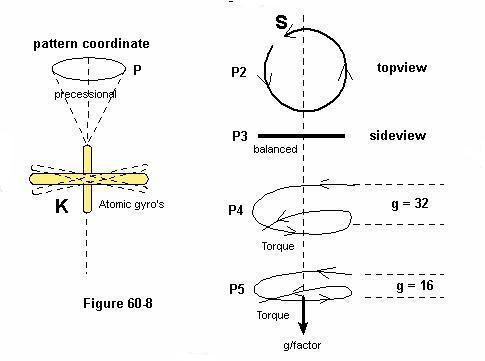1. You must forgive me for this that my only real problem here is to find the words to express my thoughts.  That oval at P therefore is in fact a circle noted P2 in a top view of it, and it is P3 in a side view of it.

2. That circle however is not a plate, nor a wheel, or a washer, - as it may appear, - but rather the pattern in which the movement that is called "its coordinate" proceeds

3. Then as a torque is placed upon the mass at K, the coordinate movement changes in this manner, at P2 - the leading end S, dips under so that now instead of a flat circle it takes on the resemblance of a screw as shown by P4, and P5.  Additionally I demonstrated the same in figure 60-3, by the picture of the screw propeller.

4. The factor of gravity may then be illustrated here in the torque by the degree thereof. At P4, the strength of the force being greatest shows itself by the figure of 32, which is in lb.  While at much higher altitudes, the g/factor having been reduced to 16 (in lb) the torques is seen to be equally less.

5. This then is accurate, for if from this, one construes that at a g/factor of 32 the rate of acceleration is 32 ft/sec/sec, while at 16 the rate of acceleration is at 16 ft/sec/sec, he is quite correct.

6. A comparison is like a nut on a long threaded bolt, by ten threads per inch the downward speed is greater than by 20 threads per square inch.

REMINDER

1. After all now is said and done, someone will reason or assert that the gravity by the rotation of the earth is inborn with the amount of g shown upon the scale as the gross outcome. But that person has not read my writing, and that reasoning is shortsighted in the fact that the scale shows the same degree of gravity upon the equator as it does upon the poles.

2. And it is shortsighted in this that there are indeed two separate and distinct vectors from which all substance on the earth is drawn, wherefore the g upon the scales cannot in itself be the full value of g by which anything upon the earth is drawn.

3. And thirdly, that very same factor of g, that centers on the earth axis, neither shows up in the horizontal when a mass is accelerated, or brought into a change of direction, it being irrelevant to the factor of g by the rotation of the earth.

4. Nor is magnetic force such a simple structure as it is usually presented. It is correct to show it as a figure of eight passing through all the earth, but that also is elementary in this that such is the greater overall pattern of it. For in coming one step closer to its fundamentals you will find that the movement coming out of the north end of the mass is in two directions, in as well as out.

5. And as by the illustration Fig 60-W, the figure eight of force is not proceeding in or out at the polar ends, though they are centered there. This is also - one reason - why atoms have multiple points at which to adhere.

1. A clear demonstration of this is with our sun whereupon the pattern of the magnetic lines are clearly seen in the flares that bulge upwards due to gaseous pressures, and how for their U shape they are seen upon any location of the sun.

2. Anyone having understood the beauty of the priceless gems that I have put on display, ought to realize that there is no such thing as gravity waves. By waves we understand a coordinate such as light and radio signals, or those of x-rays and the like.

3. The coordinate by which the gravitational movement is implemented, is for lengths thereof hardly a wave, for only in its whole, in its figure of eight may it be referred to as a wave, (but not rightly).

4. The gravitational wave then as such, if we are determined to invent a term for it, is in fact that coordinate instigated by the torque upon the fundamental components of the inertial mass. And as such it is in error to classify it by the term wave, as it should be, and in fact is, a coordinate, a pattern of movement distinct from the standard of a wave.

5. We may just as well forget about looking for any gravitational waves, since man will never find them. He should rather look into inertia and into its precession to find the coordinate that forces the mass of any size or quantity down at a single relative velocity. And that said velocity will decrease in feet per unit of time as the factor of g decreases.

6. For again as soon as any mass comes to a radius where the local factor of g has come to a zero, or a minus of, it will no longer fall, (by gravity). If therefore its movement is at a constant velocity in an orbital path, it will remain there.  An increase or decrease in its velocity will then be the factor to bring it out of orbit, and relevant to other factors.

FACTOR OF INERTIA

1. In order to enhance our understanding of that factor of gravity upon our earth that is also called a "factor on inertia" we ought to review some examples.

2. By figure 60-9, it is obvious and common knowledge that the 32.174 (in lb) at sea level is a degree of force by gravitational pull, like at point A, in the illustration all things are pulled with that degree of force.  But that force is not only a force it can also be called a degree of resistance.

3. If per example we act sideways to the direction of gravity as by B, in the illustration, not in any way at all moving towards nor away from any gravitational pull, our movement is resisted by a factor of force that is equal to the gravitational pull, even though it has nothing at all to do with gravity in its direction thereof.

4. When for another example illustrated as C, we take a racecar or the like to drive in a circle around the track, our movement into the change of direction is also again resisted by exactly the same amount of force that we spell out for gravity.

5. Or when by D, we effect ourselves at some greater speed around mother earth, we are again subject to the same factor of resistance that we established as a factor of gravity, at sea level being 32.174 (in lb)

6. Take note of this how I do not list this factor, as a number of in pounds of weight, but rather as simply a number in a factor of it.  The reason that I add to say (in lb) is because it can also be expressed in kilograms.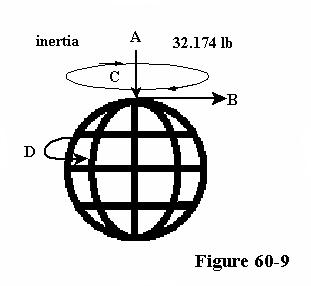1. Seeing therefore how that so called factor of gravity is equally the same for any and all mass moving into any direction other than gravity, and how it resists any change in direction irrelevant of any gravity, it becomes obvious how that factor is more prominently one of "inertia" than being one of gravity.  And how very correct that is

2. Shall we then conclude that it is the magnetic force to effect that degree of inertia upon the mass, how it is by gravitational pull that all mass comes to abide by that factor of inertia, or resistance as we might also say?

3. The answer is a no, with some explanation into a yes as well.  The fact of the matter is just the other way around, since it is foremost a factor of inertia, a factor relating to and of the angular momentum (spin) of all atoms, together with any linear movement that may be placed upon them.

4. Accordingly, and I will make this statement first; how that inertia -- is its inertia regardless of any gravitational pull or implication. Yet then we come to the next question namely; how and by what that inertia came to its numerical value of 32.174 (in lb) (at sea level)?

5. Here is where the magnetic force of the earth steps in to by its power effect that numerical value.  So it is that I pronounced a no, as well as a yes at our previous question, to realize that inertia is inertia and foremost to keep any and all mass in place and/or in direction of, while the factor of it - expressed numerical for a strength thereof is by the earth's magnetic entity.

6. Therefore also when we gain altitude, and none of the atoms slowed down to present any lesser degree of angular moment, to as we might say decrease their inertial value, yet the overall outcome of their inertial value decreased in a factor thereof since it was placed further from the center of its magnetic entity effecting its numerical value in the overall scope thereof.

7. Note how I used the term "overall" to clarify that none of the atoms in their fundamental momentum decreased in any wise at all, but rather the torque factor upon their (so called) precessional movement decreased, for there it is where the 3M places its hold in whatever value it may be relevant to speed and radius.

8. When therefore we have a racetrack at sea level the effect in the centrifugal for the change into the direction by which the cars race around a circular track must be computed by the figure of 32.174.

9. If on the other hand that racetrack were up in the mountains a mile or so above sea level, where the factor of gravity (just for the example) had decreased to 31.9, our computations should be accordingly.

10. Curious thus how high up in the mountains as well as at seal level the atoms in their momentum have not changed, and why then should there be any smaller numerical figure by which to calculate the centrifugal resistance by which the cars race around the track?

11. Inertia after all is inertia all the same when it is founded upon the angular momentum of the mass.  Should we therefore high up in the mountains adjust our calculations to the lower gravitational factor, or remain with our sea level figure?

12. Frankly speaking we do not have a set figure of the inertia of any mass as such - outside of what we obtained by gravitational means. My judgment in this matter is; if our movement high up in the mountains is either up or down, or vectored to earth's axis, we must utilize that factor of gravity pertaining to the altitude.

13. If on the other hand our movement is not in any gravitational direction nor vectored to earth axis, like cars going around a racetrack, or a vehicle coming to a stop, or accelerating, we might best use our sea level figure, as if that figure were the fundamental figure of it.

14. And yes I know that it is indeed curious how - if so fundamental - that it happens to be exactly the same as that force by which the magnetic entity of the earth draws on all mass at seal level.

15. If for any reason I am in error here, we will have to blame Sir Isaac Newton along with me, since he made the law after that figure, to at all times high up above the earth where the factors of gravity are diminished still use the sea level weight figure in our computations.

ATOMIC GYRO.

1. I think it odd that man never got around to looking at an atom in its behavior as a gyro, nor did I ever hear him speak of coordinates. Yet in all this he invented a myriad of waves to do all sort's of things, and he speaks of chemicals in which waves are captivated or recognized.

2. How then did it not dawn on him how each chemical has its specific coordinates, and that it are coordinates, which in all respects are movements by design?

3. Perhaps he is so obsessed with the atom as a planetary system, the likes unto our solar system, that he is unable to see the forest for its trees.  I do not discount the fact that there is movement within and upon the atom, nor really for parts of it, but if they be as man has - is left to question.

4. His invention of charges in the opposites has however clouded his mind to any reality in the nature of the atom.  For any part of nature, however fundamental, it is utterly impossible to have - as man claims - a single charge, for that means there must be a single sided coin, an ideal that is in violation of any and all laws of motion as well as of nature.

5. And for any atom to shed any of its parts (so called electrons), then to continue in its own nature, violates all laws of nature. The atomic bomb in part demonstrates that by taking away any parts of an atom, stable or otherwise, comes to disrupt it altogether.

6. Taking a single -so called electron from the Oxygen atom, turns it into Nitrogen, how then does the water remain water when electrified?

7.  And why did man invent so much open space in the atom from its core to the outer edges? Was it because in looking at our solar system there was more empty space than planets?

8. And how will man possibly behold that obvious angular momentum of the atom?  Do we behold the blades of a propeller turning at high speed?

9. Yes but, so one might answer; "We did see a core at the center of that atom.  And yes I concur, for even so one can see the hub of a propeller when it blades are not seen. If nothing else perhaps I might have convinced some that we have yet to learn a great deal about the atom and its operation in the scheme of things, and how to measure its volume.

10. The fact that each separate element has its specific coordinate, is not to say that the same is a product in the material sense, but rather in the coordinate movement of the material parts.

11. Wherefore this being so, that atom for its movement all in all, is more than just a complicated piece of machinery, and astonishing to us not having a full, or even a partial knowledge of in the how and why of these coordinates.

12. The scientists may have it all figured out, for levels, and photons, the degree of charges, and forces for the strong and weak, or what all these may have dreamed up for it.  I for one am as yet at a loss to define the atoms in their many distinct coordinates, as well as the reality of its parts.

13. When we consider light in its wavelength and frequency, how many wavelets do you think it takes for us to behold that which we see?

14. If no more than 1% of all the wavelets came to us we would see perfectly. Consider how the light in our dwellings is turned off and on sixty times each second, and yet to us it appears continuous.

15. Then consider how light in its velocity of 300.000 km/sec comes to three hundred billion centimeters, and how each centimeter is at least a billion angstroms, while the longest of wavelets by which to see is but 7000 angstroms in length.

16. When therefore you compute how many in but 1% may come to you, it is more than a hundred thousand, a far cry from 60 per second, by which the light already becomes continuous to us.

17. Like our earth in size is to the whole of the universe - so there is more of nature which we do not know - than what we do know.

18. Take any cube of air before you and consider how at all times simultaneously therein pass many telephone conversations, radio sounds, television in its many channels, light, along with the sound of voices, all passing upon that flexible gaseous substance  that by itself moves as in wind.

19. How phenomenal even that simple part of nature is, to conduct all these things with perfection.

20. Then as we consider a piece of wood, or metal, or the finger upon our hand, the astronomical number of atoms it must contain, and how these atoms hold on to one another by nothing more than movement, and themselves producing movement, and all the many coordinates by which it holds shape and relates to everything else in nature.

21. What a marvel of engineering this nature is, and how grandiose He must be that made all this nature in such endless quantities.

22. When a man comes to understand nature in a part thereof, he comes to appreciate and wonder at the Maker thereof.

23. Whoever then considers not the Lord the Almighty Creator - and does not consider His works, is beastly ignorant, yes my friend -- beastly ignorant.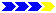Next page